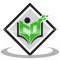## Relation between Work and EnergyUpdated on 21-Dec-2022 15:34:26
Introduction The energy of the machinery body can be transferred to other objects to move. Transferring the energy to other body particles is in the form of force. The actual amount of energy that is transferred by the help of inside and outside forces to move to another object is considered as work as well as work done. Therefore, the relation between the work and energy in physics is direct and the difference between these two factors can be seen in the kinetic energy of a particular object that is work done by the specific object. Relation between Work and ... Read More

## Derivation of Work Energy TheoremUpdated on 13-Oct-2022 11:19:47
Introduction The Work-Energy Theorem outlines that the net completed work by the forces that are applied to the machinery body from external sources that is equivalent to the alternation rate of the kinetic energy of a particular object. The Work-Energy Theorem on an object is done by all of the forces rather than applied to a single force. According to the Work-Energy Theorem, the kinetic energy is mainly defined as the required work power for accelerating the object from the resting phase to a kind of state that required velocity. What is the work-energy theorem? Figure 1: Diagram of work-energy ... Read More

## Conservation of Mechanical EnergyUpdated on 13-Oct-2022 11:19:47
Mechanical Energy Mechanical energy is the sum of kinetic energy and potential energy in an object that is used to do a particular work. In other words, it describes the energy of an object because of its motion or position, or both. Consider the case of an ideal simple pendulum (friction-less). We can say that this system's mechanical energy is a combination of its kinetic and gravitational potential energy. As the pendulum swings back and forth, there is a constant exchange of kinetic and potential energy. When the bob reaches its maximum height, the system's potential energy is the highest, ... Read More